Vibroengineering PROCEDIA

Published: 18 May 2023

# Investigation on the flow-induced tangential force characteristic of labyrinth seals under different operation parameters

Qianlei Gu1
Jiangang Yang2
1, 2School of Energy and Environment, Southeast University, Nanjing, China
Corresponding Author:
Jiangang Yang
Views 8

#### Abstract

The flow-induced tangential force ${F}_{t}$ is crucial for the annular gas seal stability. In this paper, a three-dimensional CFD model is established to study the ${F}_{t}$ characteristics of the labyrinth seal. The overall ${F}_{t}$ of the seal section is decomposed into each seal cavity. The ${F}_{t}$ characteristic of each seal cavity versus rotation speed $\omega$ and inlet pressure ${p}_{in}$ is analyzed. The result shows that the sign of ${F}_{t}$ in each seal cavity changes from the negative to the positive along the leakage direction, resulting in a specific crossing tooth number ${N}_{c}$ at which 0 N. When the tooth number is smaller than ${N}_{c}$, the seal stability increases with the increase of $\omega$ and ${p}_{in}$. When the tooth number is larger than ${N}_{c}$, the trend is reversed. When the tooth number equals ${N}_{c}$, the seal stability is in a critical state, showing no dependency to the $\omega$ and ${p}_{in}$. The ${N}_{c}$ is a novel index for the labyrinth seal stability.

## 1. Introduction

Annular gas seals are key components in turbomachinery to restrict leakage from high-pressure regimes to low-pressure regimes . Its sealing performance direct impacts the unit efficiency. With the development of the modern turbomachinery, the flow-induced vibration caused by seals becomes prominent [2, 3]. The research on the seal stability is important for the stable operation of turbomachinery.

Labyrinth seals (LS) are widely used in turbine machinery due to their low cost, simplicity, and reliability . Extensive research has been conducted on their dynamic performance. Wu and San Andrés  compared the rotordynamic force coefficients of a teeth-on-stator LS and an interlocking LS. The result shows that when compared to the interlocking LS, the teeth-on-stator type has a larger effective damping coefficient for operation with whirl frequency up to 30 % above the synchronous rotor speed. Zhang et al.  numerically studied the impact of inlet preswirl on the rotordynamic performance of three types of LSs. They found that negative preswirl is beneficial to the stability of LSs. Zhang and Gu  proposed a novel anti-stagnation LS with micro-nozzles on adjacent seal teeth. The high-speed jet, driven by the intrinsic pressure difference in the seal domain, is injected into the seal cavity with the direction opposed to the circumferential flow. With controlled circumferential flow, the effective damping coefficient is effectively improved. Sun et al.  experimentally studied the impact of a swirl brake on the dynamic performance of LS. The results indicate that the swirl brake effectively reduces the seal swirl ratio, thereby decreasing the destabilizing forces of the seal. Li et al.  numerically investigated the effects of operational conditions on the dynamic performance of a teeth-on-stator LS. They found that the magnitudes of all seal force coefficients are roughly proportional to the seal supply pressure but insensitive to the backpressure. Increasing rotation speed and inlet preswirl both result in a significant decrease in effective damping.

The above researches mostly focus on the overall dynamic characteristics of LSs. However, the flow field parameters such as pressure, temperature, velocity, and turbulence intensity vary greatly along the leakage direction. The impact of the flow field parameter differences along the leakage direction on the seal stability is unclear.

In this paper, a three-dimensional numerical model is established to study the flow-induced tangential force ${F}_{t}$ characteristics of the LS. The overall ${F}_{t}$ of the seal is decomposed into each seal cavity. The ${F}_{t}$ characteristic of each cavity versus rotation speed and inlet pressure is analyzed. Based on the ${F}_{t}$ characteristics, the conception of the crossing tooth number ${N}_{c}$ is proposed as a novel stability index for the labyrinth seal.

## 2. Numerical method

Fig. 1 shows the schematic diagram of the studied labyrinth seal. Table 1 provides the detailed seal dimensions. The rotor’s eccentric direction aligns with the positive direction of the $y$-axis. When the ${F}_{t}$ is in the same direction with the rotor velocity, it is considered a positive value. Conversely, when the force and rotor velocity are in opposite directions, the ${F}_{t}$ is considered a negative value. To ensure seal stability, a negative ${F}_{t}$ is desired since it removes energy from the forward whirl motion.

Fig. 1Schematic diagram of the studied labyrinth seal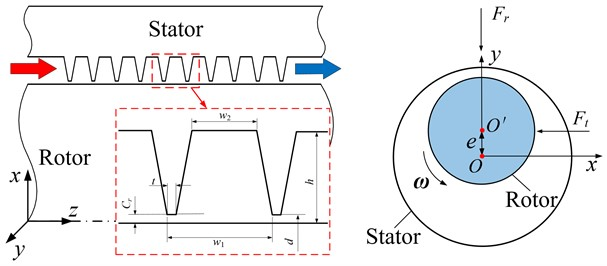Table 1Seal dimensions

 Parameter Value Diameter $d$ 60 mm Clearance ${C}_{r}$ 0.2 mm Pitch ${w}_{1}$ 3.8 mm Cavity height $h$ 3.5 mm Tooth thickness $t$ 0.25 mm Width of cavity top ${w}_{2}$ 2.3 mm Eccentric value $e$ 0.01 mm

To solve the Reynolds-averaged Navier-Stokes equations, the commercial CFD software ANSYS CFX was used. At the inlet boundary, the total pressure and temperature were defined, while the average static pressure was specified at the outlet. The turbulence model employed was the standard $k$-$\epsilon$, with a turbulence intensity of 5 %. The walls of both the rotor and stator were defined as nonslip, and the scalable wall function was applied to control the value of $y$+ within 40-100. The target for convergence was set such that the root mean square residuals of the momentum equations, mass equations, energy equations, and turbulence equations were below 10-6 [10, 11]. Table 2 provides the operational parameters for the seals.

Mesh independence studies are performed for the 10-tooth case (8.9 bar, 60 krpm). The ${F}_{t}$ is chosen as the evaluation parameter. As shown in Table 3, the ${F}_{t}$ of the studied seal shows insensitivity to the increasing mesh density when the element number is larger than 2.4×106 (the difference is within 1.5 % compared with the 5.6×106 case). The mesh density of 2.4×106 elements can meet the mesh independence. Fig. 2 shows final mesh model adopt for the study.

Table 2Operation parameters

 Parameter Value Inlet temperature ${T}_{in}$ 287 K Inlet pressure ${p}_{in}$ 2.9~8.9 bar Outlet pressure ${p}_{out}$ 1 bar Inlet preswirl ${v}_{0}$ 0 m/s Rotation speed $\omega$ 0~60 krpm

Table 3Mesh independence study

 Mesh elements ${F}_{t}$ (N) Difference (%) 8×105 –0.109 48.1 1.6×106 –0.181 13.8 2.4×106 –0.207 1.4 5.6×106 –0.210 0 (reference)

Fig. 2Final mesh model adopt for the study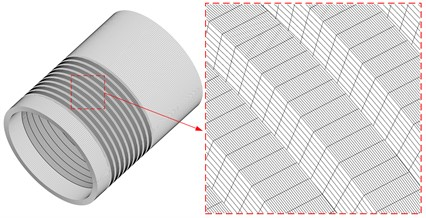## 3. Results and discussions

Fig. 3 shows the trend of total flow-induced tangential force ${F}_{t}$ versus rotation speed $\omega$ (2.9 bar). The tooth number ranging from 5 to 20 is studied. It can be seen that rotor rotation is the main cause of ${F}_{t}$. As the $\omega$ increase, the ${F}_{t}$ magnitude of studied seals all presents an increase trend. As the tooth number increases, the direction of ${F}_{t}$ changes from the negative to the positive. For the 5-tooth and 10-tooth cases, the ${F}_{t}$ presents the opposite direction to the rotation and the stability increases as the $\omega$ increases. In contrast, for the 15-tooth and 20-tooth cases, the ${F}_{t}$ is in the same direction of rotation. The seal stability of 15-tooth and 20-tooth LSs decreases as the rotation speed increases. Notably, for the 14-tooth case, ${F}_{t}$ is close to zero at all studied $\omega$.

Fig. 4 shows the trend of total flow-induced tangential force ${F}_{t}$ versus inlet pressure ${p}_{in}$ ($\omega =$ 60 krpm). Similar to the trend of ${F}_{t}$ versus $\omega$ shown in Fig. 3, the ${F}_{t}$ magnitude of the studied seals also presents an increase trend as the ${p}_{in}$ increase. The ${F}_{t}$ of the 5-tooth and 10-tooth cases present the opposite direction to the rotation, and the stability increases as the ${p}_{in}$ increases. The ${F}_{t}$ of the 15-tooth and 20-tooth cases present the same direction to the rotation and the stability decreases as the ${p}_{in}$ increases. Similar to the result show in Fig. 3, the ${F}_{t}$ of the 14-tooth case is also close to 0 N at all the studied ${p}_{in}$, showing no dependency to the ${p}_{in}$. This phenomenon will be explained in the following section.

It can be seen that there is a crossing tooth number ${N}_{c}$ for the LS. When the tooth number is less than ${N}_{c}$, the system stability is positively correlated with the increasing $\omega$ and ${p}_{in}$. When the tooth number is greater than ${N}_{c}$, the system stability is negatively correlated with the increasing $\omega$ and ${p}_{in}$, and the larger the tooth number, the worse the seal stability. When the tooth number is equal to ${N}_{c}$, the total ${F}_{t}$ is close to 0 N, showing no dependency to the $\omega$ and ${p}_{in}$. Thus, the labyrinth seal system with different tooth numbers can be divided into the stable and unstable state based on the crossing tooth number ${N}_{c}$. Combining the results from Fig. 3 and Fig. 4, it can be concluded that the ${N}_{c}$ of this model is about 14. The 5-tooth and 10-tooth cases are at the stable states, while the 15-tooth and 20-tooth cases are at the unstable state. The 14-tooth case is at the critical stable state.

Fig. 3Total flow-induced tangential force Ft versus rotation speed ω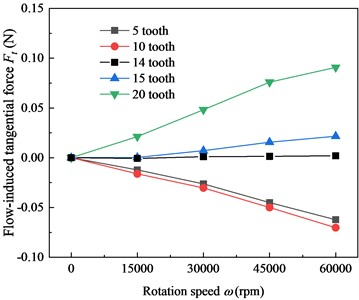Fig. 4Total flow-induced tangential force Ft versus inlet pressure pin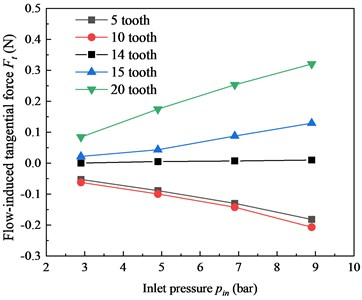The above research shows that the total ${F}_{t}$ has a close relationship with the tooth number of the LS. For reveal the ${F}_{t}$ component, the overall ${F}_{t}$ of the seal section is decomposed into each seal cavity. The ${F}_{t}$ characteristic of each seal cavity versus rotation speed $\omega$ and inlet pressure ${p}_{in}$ is analyzed. Fig. 5 shows the ${F}_{t}$ component of each seal cavity under different $\omega$ for 10-tooth and 20-tooth cases (2.9 bar). Along the leakage direction, the ${F}_{t}$ of the seal cavity changes from the negative to the positive. For the 10-tooth case, the negative ${F}_{t}$ dominates seal section, and the ${F}_{t}$ magnitude increases as the $\omega$ increases. This is the reason why the magnitude of the total ${F}_{t}$ (negative value) presents the increases trend as the $\omega$ increases shown in Fig. 3. In contrast, for the 20-tooth case, the positive ${F}_{t}$ dominates seal section. This explains the reverse trend of the 20-tooth case shown in Fig. 3.

Fig. 5Flow-induced tangential force Ft distribution in seal cavities under different ω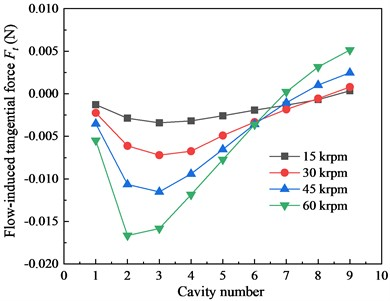a) 10-tooth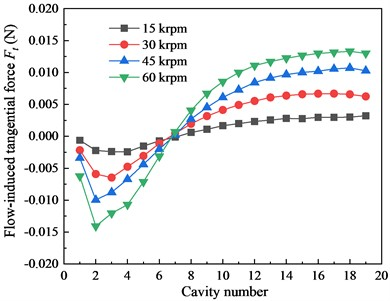b) 20-tooth

Fig. 6 shows the ${F}_{t}$ component of each seal cavity under different inlet pressure ${p}_{in}$ for 10-tooth and 20-tooth cases (60 krpm). The effect of inlet pressure is similar to that of the rotation speed. As the ${p}_{in}$ increases, the magnitude of negative ${F}_{t}$ in each cavity of the 10-tooth case continue to increase. The seal stability is enhanced. For the 20-tooth case, the positive ${F}_{t}$ is the main part. Thus, the increasing ${p}_{in}$ exerts the negative impact on the seal stability for the 20-tooth LS. The above phenomenon coincides with the results shown in Fig. 4. According to the ${F}_{t}$ distribution in seal cavities of the 10-tooth and 20-tooth cases, when the tooth number equals to the 14, the negative and positive ${F}_{t}$ of seal cavities cancel each other. This is the reason why the total ${F}_{t}$ of the 14-tooth case shows no dependency to the $\omega$ and ${p}_{in}$ as shown in Fig. 3 and Fig. 4.

Fig. 6Flow-induced tangential force Ft distribution in seal cavities under different pin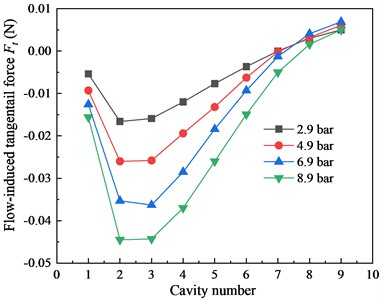a) 10-tooth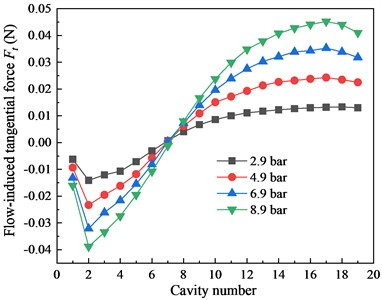b) 20-tooth

## 4. Conclusions

In this paper, a three-dimensional CFD model is established to study the flow-induced tangential force ${F}_{t}$ characteristics for the labyrinth seals. The overall ${F}_{t}$ of the seal section is decomposed into each seal cavity. The ${F}_{t}$ characteristic of each seal cavity versus rotation speed and inlet pressure is analyzed. Conclusions are summarized as follows:

1. The total ${F}_{t}$ of the labyrinth seal is approximately proportional to the seal supply pressure and rotation speed.

2. The direction of the ${F}_{t}$ in the seal cavities changes from positive (near the inlet section) to negative (near the outlet section) along the leakage direction. For labyrinth seals with a small number of teeth, the negative ${F}_{t}$ dominates the seal cavities. For those with a large number of seal tooth, the positive ${F}_{t}$ plays a more significant role in seal cavities.

3. The labyrinth seal has a crossing tooth number ${N}_{c}$. When the tooth number is smaller than ${N}_{c}$, the ${F}_{t}$ is always negative, and the seal stability increases with an increase in rotation speed and inlet pressure. When the tooth number is larger than ${N}_{c}$, the ${F}_{t}$ is always positive, and the seal stability is negatively correlated with an increase in rotation speed and inlet pressure. When the tooth number equals ${N}_{c}$, the seal stability is in a critical state, showing no dependency on rotation speed and inlet pressure. ${N}_{c}$ is a novel index for evaluating labyrinth seal stability.

#### Acknowledgements

The authors are grateful for the Grants from the National Natural Science Foundation of China (52075096).